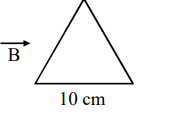# A coil in the shape of an equilateral triangle of sideQuestion:

A coil in the shape of an equilateral triangle of side $10 \mathrm{~cm}$ lies in a vertical plane between the pole pieces of permanent magnet producing a horizontal magnetic field $20 \mathrm{mT}$. The torque acting on the coil when a current of $0.2 \mathrm{~A}$ is passed through it and its plane becomes parallel to the magnetic field will be $\sqrt{\mathrm{x}} \times 10^{-5} \mathrm{Nm}$. The value of $\mathrm{x}$ is..........

Solution:$\vec{\tau}=\overrightarrow{\mathrm{M}} \times \overrightarrow{\mathrm{B}}=\mathrm{MB} \sin 90^{\circ}$

$=\mathrm{MB}=\frac{\mathrm{i} \sqrt{3} \ell^{2}}{4} \mathrm{~B}$

$=\sqrt{3} \times 10^{-5} \mathrm{~N}-\mathrm{m}$

Ans. 3